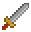# Colliders

Colliders are abstractions over geometry in Excalibur, they implement the Collider interface and know how to detect intersecions with other colliders, test ray casts, check point containment, etc. Related but not the same are bodies which are abstractions over the collision response

Colliders attached to an Entity will have a Entity.owner populated.

Keep in mind colliders are relative to the owner TransformComponent, they only represent geometry relative to an entity TransformComponent. Colliders can be shifted using the Collider.offset.

Colliders don't have a position in the world without an Actor/Entity with a position

### CircleCollider

The CircleCollider defines a circular geometry and can be created and added to an actor

``````// Actors have a built in circle collider if radius is set
const actorWithCircleCollider = new ex.Actor({
pos: ex.vec(5, 5),
})

// Alternatively you can define and set a collider yourself

const circle = new ex.CircleCollider({
})

// or

const circle = ex.Shape.Circle(10) // 10 pixel radius

const actor = new ex.Actor({
pos: ex.vec(100, 100),
collider: circle,
})

// Change the collider afterwards
actor.collider.set(circle)``````

### Box and PolygonCollider

The PolygonCollider allows the definition of arbitrary convex polygons. Convex meaning there are no dents in the shape, for example "Pac-Man" would be a non-convex shape.

A box collider is created using the PolygonCollider, there is a Shape.Box helper to build these.

``````// Actors have a built in box collider if width/height are set
const actorWithBoxCollider = new ex.Actor({
pos: ex.vec(100, 100),
width: 100,
height: 10,
})

// Alternatively you can define and set a collider yourself
const box = ex.Shape.Box(100, 10)

const actor = new ex.Actor({
pos: ex.vec(100, 100),
collider: box,
})``````

A polygon collider can be defined using a set of points specified in clockwise order (also known as "winding").

``````const triangle = new ex.PolygonCollider({
points: [ex.vec(-100, 0), ex.vec(0, -50), ex.vec(100, 0)],
})``````

### EdgeCollider

An EdgeCollider can be used to define a single line collider.

They are useful for creating walls, barriers, or platforms in your game.

Edge points are relative to the Entity position, so begin of `(0, 0)` means it starts right on the top of the Entity TransformComponent

``````const edge = new ex.EdgeCollider({
begin: ex.vec(0, 0),
end: ex.vec(100, 0),
})

const actor = new ex.Actor({
pos: ex.vec(100, 100),
collider: edge,
})``````

### CompositeCollider

CompositeCollider can be used to attach multiple colliders to an entity at once. This can be useful when generating complex shapes, like for example a capsule collider for a player Actor.

``````// Capsule Collider
const capsule = new ex.CompositeCollider([
ex.Shape.Circle(10, ex.vec(0, -20)),
ex.Shape.Box(20, 40),
ex.Shape.Circle(10, ex.vec(0, 20)),
])

const player = new ex.Actor({
pos: ex.vec(100, 100),
collider: capsule,
})``````
##### Get Involved